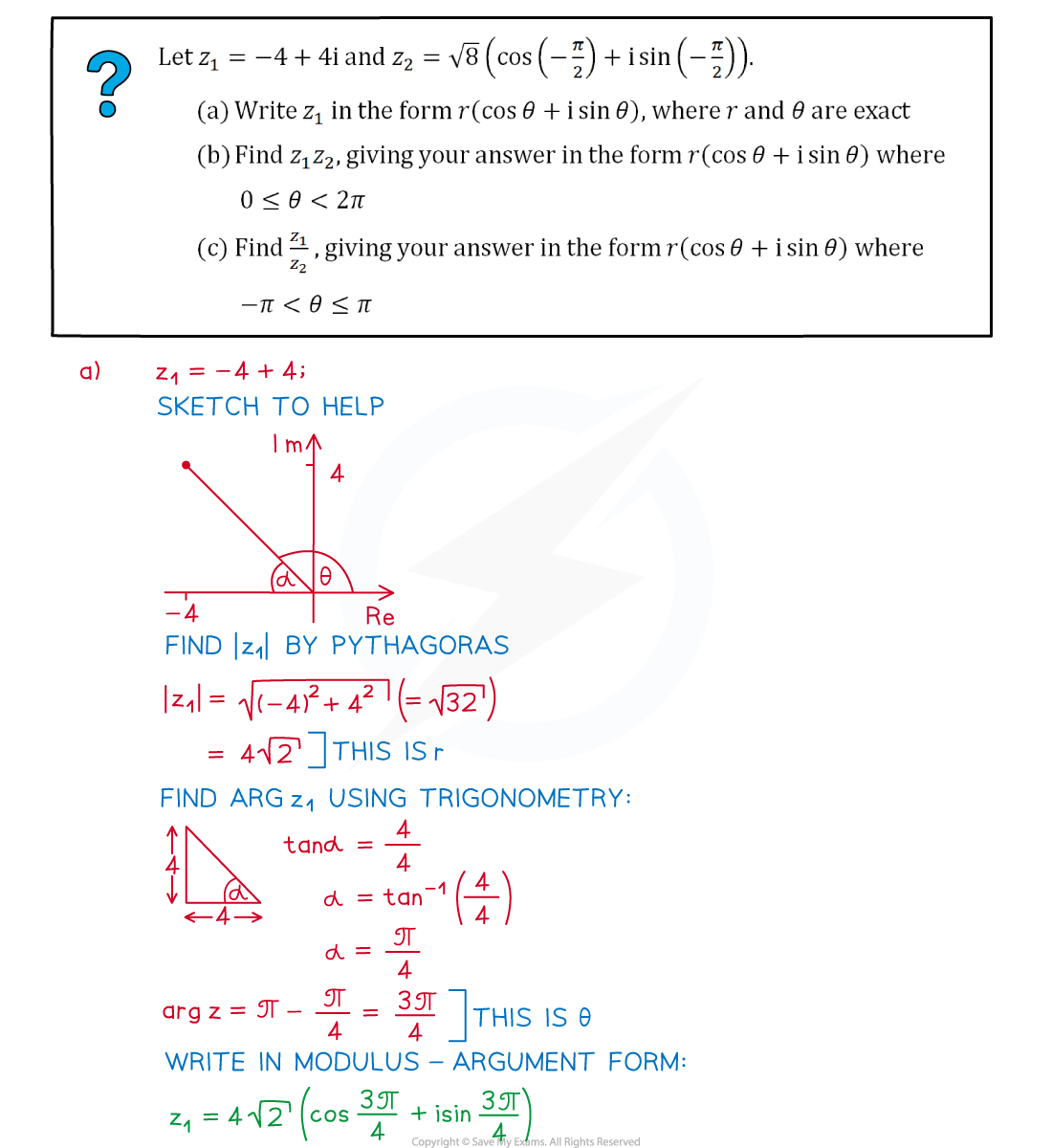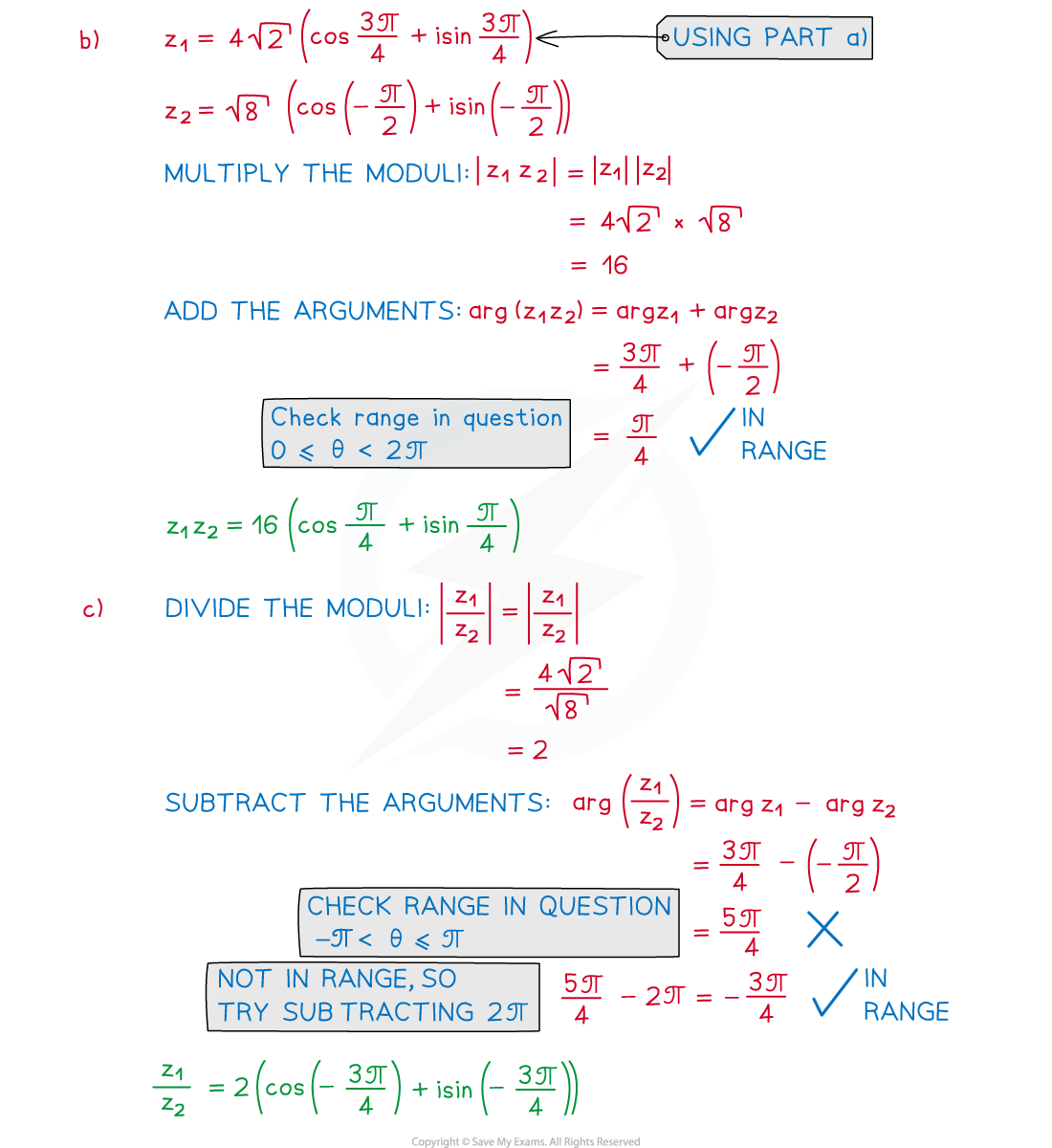# CIE A Level Maths: Pure 3复习笔记8.2.3 Modulus & Argument

### Modulus & Argument

#### How do I find the modulus of a complex number?

• The modulus of a complex number is its distance from the origin when plotted on an Argand diagram
• The modulus of  z  is written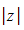• If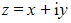, then we can use Pythagoras to show…
•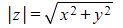• A modulus is always positive
• the modulus is related to the complex conjugate by…
•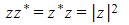• This is because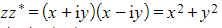• In general,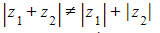• e.g. bothand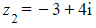have a modulus of 5, but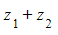simplifies to 8i which has a modulus of 8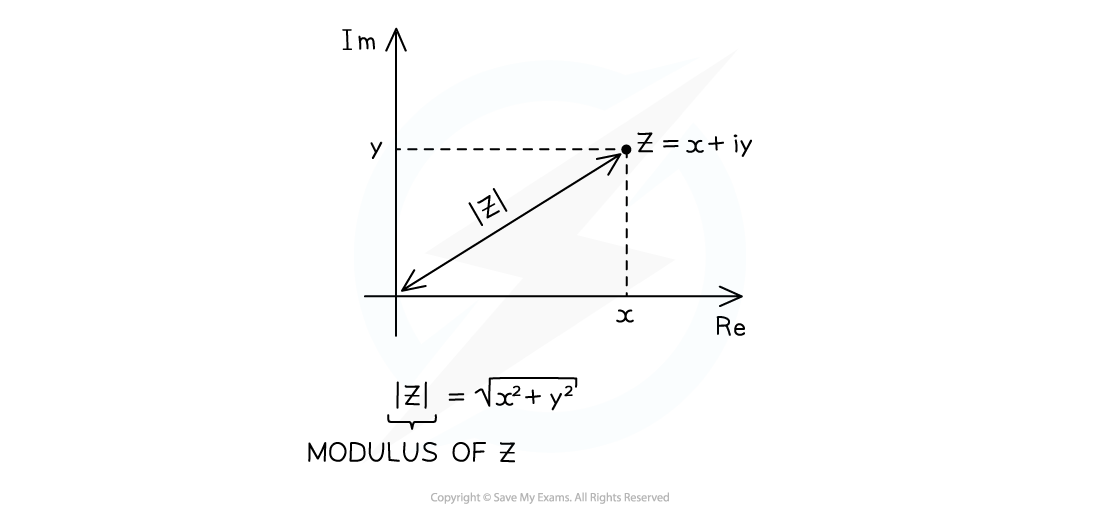#### How do I find the argument of a complex number?

• The argument of a complex number is the anti-clockwise angle that it makes when starting at the positive real axis on an Argand diagram
• Arguments are measured in radians
• Sometimes these can be given exact in terms of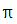• The argument of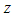is written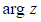•  Arguments can be calculated using right-angled trigonometry
• This involves using the tan ratio plus a sketch to decide whether it is positive/negative and acute/obtuse
• Arguments are usually given in the range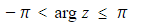• Negative arguments are for complex numbers in the third and fourth quadrants
• Occasionally you could be asked to give arguments in the range• The argument of zero, arg 0  is undefined (no angle can be drawn)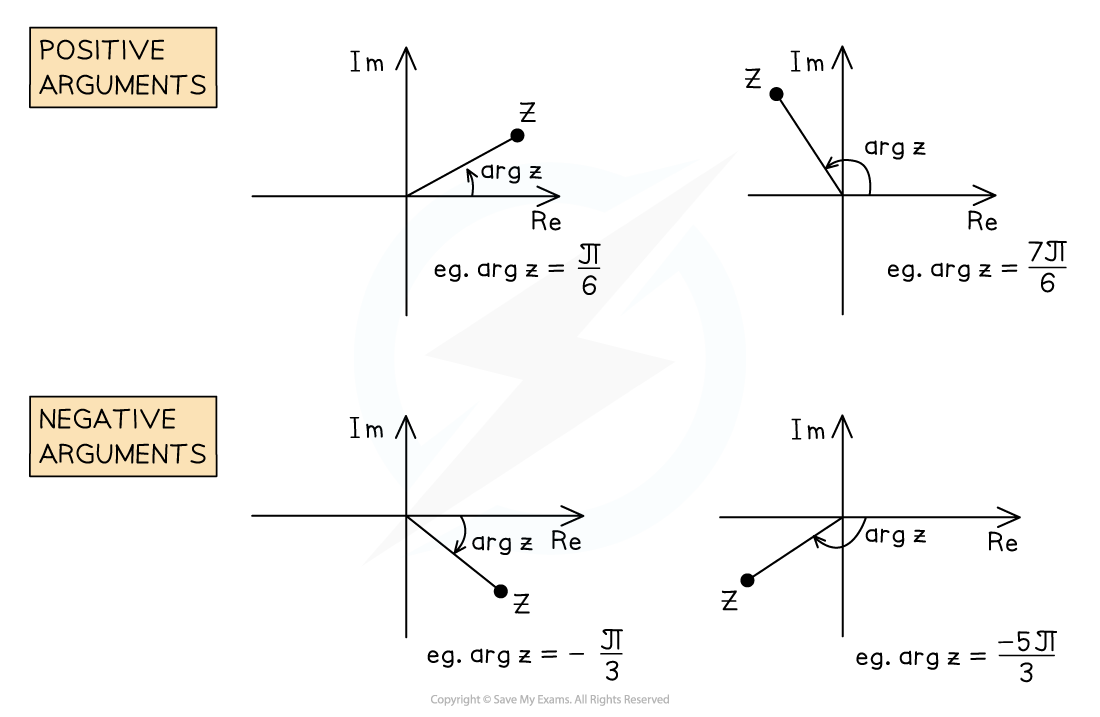#### Worked Example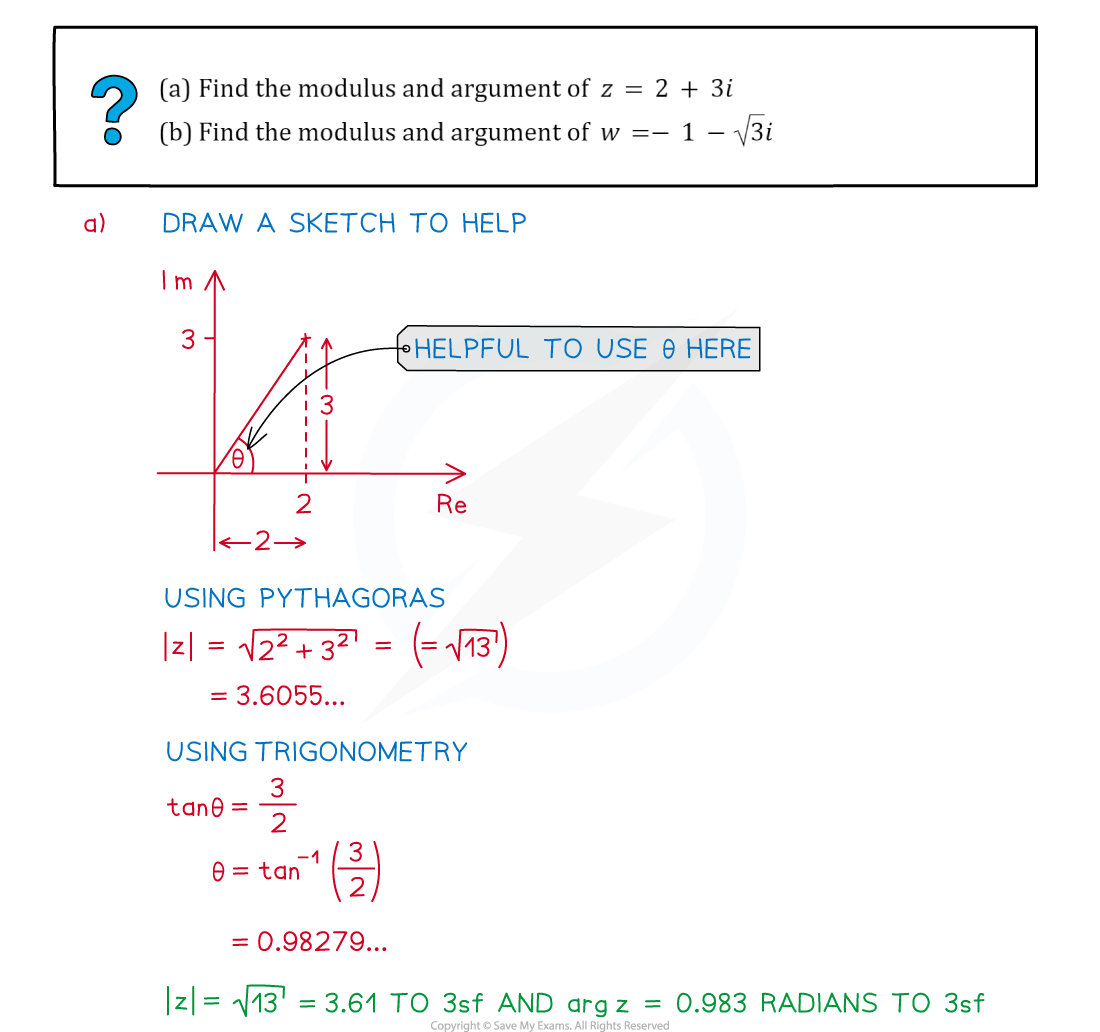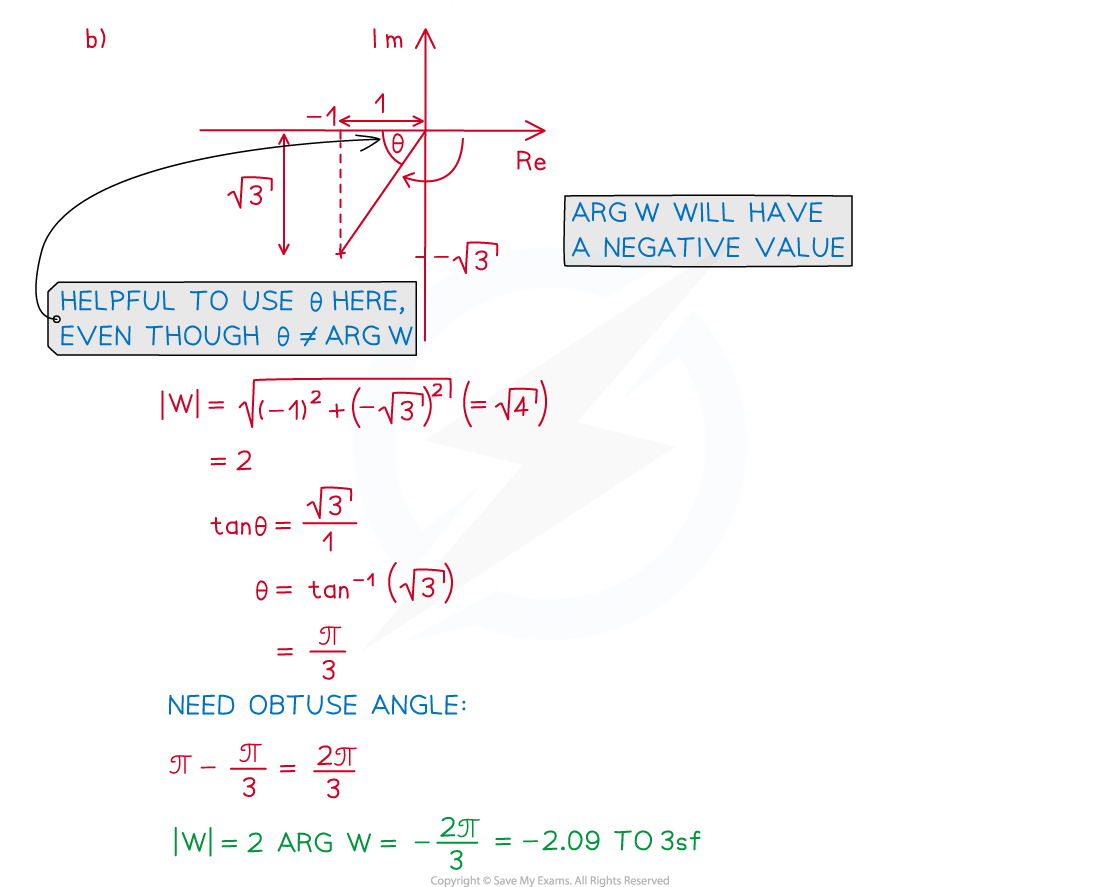#### Exam Tip

• Give non-exact arguments in radians to 3 significant figures.

### Modulus-Argument (Polar) Form

The complex number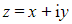is said to be in Cartesian form. There are, however, other ways to write a complex number, such as in modulus-argument (polar) form.

#### How do I write a complex number in modulus-argument (polar) form?

• The Cartesian form of a complex number,, is written in terms of its real part,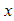, and its imaginary part,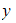• If we let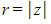and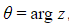, then it is possible to write a complex number in terms of its modulus, r, and its argument, Φ, called the modulus-argument (polar) form, given by...
•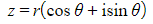• It is usual to give arguments in the range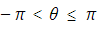• Negative arguments should be shown clearly, e.g.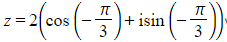without simplifying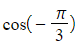to either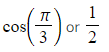• Occasionally you could be asked to give arguments in the range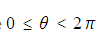• If a complex number is given in the form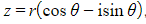, then it is not currently in modulus-argument (polar) form due to the minus sign, but can be converted as follows…
• By considering transformations of trigonometric functions, we see that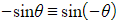and• Thereforecan be written as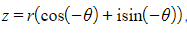, now in the correct form and indicating an argument of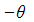• To convert from modulus-argument (polar) form back to Cartesian form, evaluate the real and imaginary parts
• E.g.becomes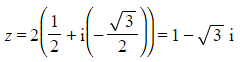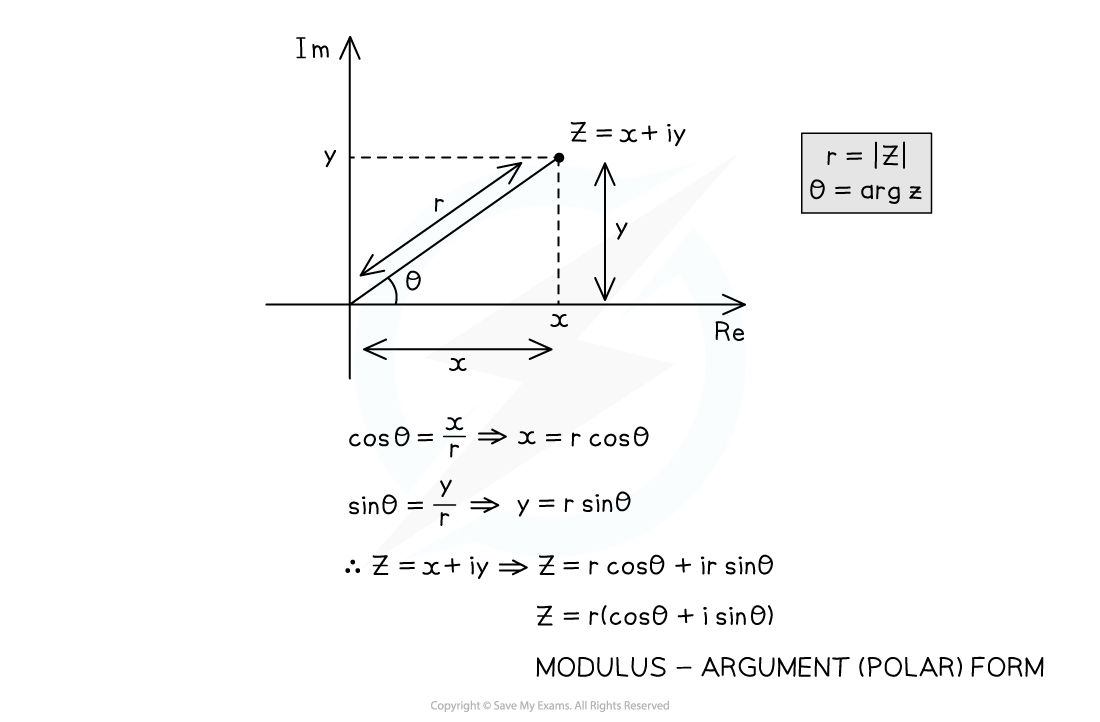#### What are the rules for moduli and arguments under multiplication and division?

• When two complex numbers,and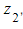are multiplied to give, their moduli are also multiplied
•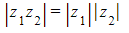• When two complex numbers,andare divided to give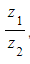, their moduli are also divided
•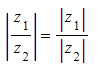• When two complex numbers,andare multiplied to give, their arguments are added
•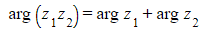• When two complex numbers,andare divided to give, their arguments are subtracted
•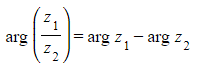#### How do I divide complex numbers in modulus-argument (polar) form?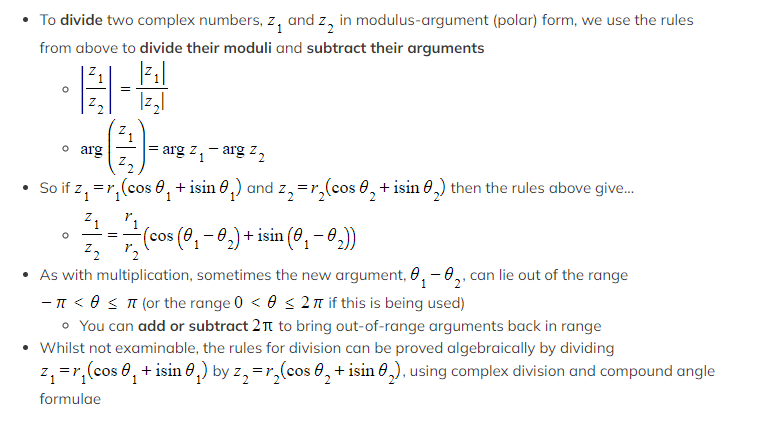#### Worked Example